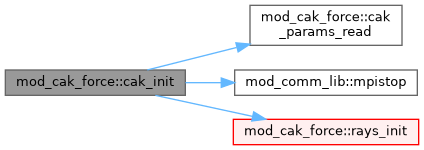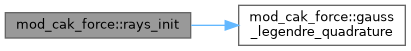MPI-AMRVAC  3.0 The MPI - Adaptive Mesh Refinement - Versatile Advection Code
mod_cak_force Module Reference

Module to include CAK radiation line force in (magneto)hydrodynamic models Computes both the force from free electrons and the force from an ensemble of lines (various possibilities for the latter). There is an option to only simulate the pure radial CAK force (with various corrections applied) as well as the full vector CAK force. Depending on the chosen option additional output are the CAK line force component(s) and, when doing a 1-D radial force, the finite disc factor. More...

## Functions/Subroutines

Public method. More...

subroutine cak_init (phys_gamma)
Initialize the module. More...

subroutine, public set_cak_force_norm (rstar, twind)
Compute some (unitless) variables for CAK force normalisation. More...

subroutine cak_add_source (qdt, ixIL, ixOL, wCT, w, x, energy, qsourcesplit, active)
w[iw]=w[iw]+qdt*S[wCT,qtC,x] where S is the source based on wCT within ixO More...

subroutine get_cak_force_radial (ixIL, ixOL, wCT, w, x, gcak)
1-D CAK line force in the Gayley line-ensemble distribution parametrisation More...

subroutine get_cak_force_vector (ixIL, ixOL, wCT, w, x, gcak)
Vector CAK line force in the Gayley line-ensemble distribution parametrisation. More...

subroutine get_gelectron (ixIL, ixOL, w, x, ge)
Compute continuum radiation force from Thomson scattering. More...

subroutine cak_get_dt (w, ixIL, ixOL, dtnew, dxD, x)
Check time step for total radiation contribution. More...

Compute velocity gradient in direction 'idir' on a non-uniform grid. More...

subroutine rays_init (ntheta_point, nphi_point)
Initialise (theta',phi') radiation angles coming from stellar disc. More...

subroutine gauss_legendre_quadrature (xlow, xhi, n, x, w)
Fast Gauss-Legendre N-point quadrature algorithm by G. Rybicki. More...

## Variables

real(8), public cak_alpha
Line-ensemble parameters in the Gayley (1995) formalism. More...

real(8), public gayley_qbar

real(8), public gayley_q0

integer cak_1d_opt
Switch to choose between the 1-D CAK line force options. More...

logical cak_split =.false.
To treat source term in split or unsplit (default) fashion. More...

logical cak_1d_force =.false.
To activate the original CAK 1-D line force computation. More...

logical cak_vector_force =.false.
To activate the vector CAK line force computation. More...

logical fix_vector_force_1d =.false.
To activate the pure radial vector CAK line force computation. More...

integer nthetaray

integer nphiray

integer gcak1_
Extra slots to store quantities in w-array. More...

integer gcak2_

integer gcak3_

integer fdf_

## Detailed Description

Module to include CAK radiation line force in (magneto)hydrodynamic models Computes both the force from free electrons and the force from an ensemble of lines (various possibilities for the latter). There is an option to only simulate the pure radial CAK force (with various corrections applied) as well as the full vector CAK force. Depending on the chosen option additional output are the CAK line force component(s) and, when doing a 1-D radial force, the finite disc factor.

USAGE:

1. Include a cak_list in the .par file and activate (m)hd_cak_force in the (m)hd_list
2. Create a mod_usr.t file for the problem with appropriate initial and boundary conditions
3. In the mod_usr.t header call the mod_cak_force module to have access to global variables from mod_cak_force, which may be handy for printing or the computation of other variables inside mod_usr.t
4. In usr_init of mod_usr.t call the set_cak_force_norm routine and pass along the stellar radius and wind temperature—this is needed to correctly compute the (initial) force normalisation inside mod_cak_force
5. Ensure that the order of calls in usr_init is similar as for test problem CAKwind_spherical_1D: first reading usr.par list; then set unit scales; then call (M)HD_activate; then call set_cak_force_norm. This order avoids an incorrect force normalisation and code crash

Developed by Florian Driessen (2022)

## Function/Subroutine Documentation

 subroutine mod_cak_force::cak_add_source ( real(8), intent(in) qdt, integer, intent(in) ixI, integer, intent(in) L, integer, intent(in) ixO, L, real(8), dimension(ixi^s,1:nw), intent(in) wCT, real(8), dimension(ixi^s,1:nw), intent(inout) w, real(8), dimension(ixi^s,1:ndim), intent(in) x, logical, intent(in) energy, logical, intent(in) qsourcesplit, logical, intent(inout) active )

w[iw]=w[iw]+qdt*S[wCT,qtC,x] where S is the source based on wCT within ixO

Definition at line 158 of file mod_cak_force.t.

Here is the call graph for this function:## ◆ cak_get_dt()

 subroutine mod_cak_force::cak_get_dt ( real(8), dimension(ixi^s,1:nw), intent(in) w, integer, intent(in) ixI, integer, intent(in) L, integer, intent(in) ixO, L, real(8), intent(inout) dtnew, real(8), intent(in) dx, real(8), intent(in) D, real(8), dimension(ixi^s,1:ndim), intent(in) x )

Check time step for total radiation contribution.

Definition at line 437 of file mod_cak_force.t.

Here is the call graph for this function:## ◆ cak_init()

 subroutine mod_cak_force::cak_init ( real(8), intent(in) phys_gamma )

Initialize the module.

Definition at line 97 of file mod_cak_force.t.

Here is the call graph for this function:subroutine mod_cak_force::cak_params_read ( character(len=*), dimension(:), intent(in) files )

Public method.

Read this module's parameters from a file

Definition at line 76 of file mod_cak_force.t.

 subroutine mod_cak_force::gauss_legendre_quadrature ( real(8), intent(in) xlow, real(8), intent(in) xhi, integer, intent(in) n, real(8), dimension(n), intent(out) x, real(8), dimension(n), intent(out) w )

Fast Gauss-Legendre N-point quadrature algorithm by G. Rybicki.

Definition at line 629 of file mod_cak_force.t.

 subroutine mod_cak_force::get_cak_force_radial ( integer, intent(in) ixI, integer, intent(in) L, integer, intent(in) ixO, L, real(8), dimension(ixi^s,1:nw), intent(in) wCT, real(8), dimension(ixi^s,1:nw), intent(inout) w, real(8), dimension(ixi^s,1:ndim), intent(in) x, real(8), dimension(ixo^s,1:3), intent(inout) gcak )

1-D CAK line force in the Gayley line-ensemble distribution parametrisation

Definition at line 213 of file mod_cak_force.t.

Here is the call graph for this function:## ◆ get_cak_force_vector()

 subroutine mod_cak_force::get_cak_force_vector ( integer, intent(in) ixI, integer, intent(in) L, integer, intent(in) ixO, L, real(8), dimension(ixi^s,1:nw), intent(in) wCT, real(8), dimension(ixi^s,1:nw), intent(inout) w, real(8), dimension(ixi^s,1:ndim), intent(in) x, real(8), dimension(ixo^s,1:3), intent(inout) gcak )

Vector CAK line force in the Gayley line-ensemble distribution parametrisation.

Definition at line 288 of file mod_cak_force.t.

Here is the call graph for this function:## ◆ get_gelectron()

 subroutine mod_cak_force::get_gelectron ( integer, intent(in) ixI, integer, intent(in) L, integer, intent(in) ixO, L, real(8), dimension(ixi^s,1:nw), intent(in) w, real(8), dimension(ixi^s,1:ndim), intent(in) x, real(8), dimension(ixo^s), intent(out) ge )

Compute continuum radiation force from Thomson scattering.

Definition at line 425 of file mod_cak_force.t.

 subroutine mod_cak_force::get_velocity_gradient ( integer, intent(in) ixI, integer, intent(in) L, integer, intent(in) ixO, L, real(8), dimension(ixi^s), intent(in) vfield, real(8), dimension(ixi^s,1:ndim), intent(in) x, integer, intent(in) idir, real(8), dimension(ixo^s), intent(out) grad_vn )

Compute velocity gradient in direction 'idir' on a non-uniform grid.

Definition at line 474 of file mod_cak_force.t.

## ◆ rays_init()

 subroutine mod_cak_force::rays_init ( integer, intent(in) ntheta_point, integer, intent(in) nphi_point )

Initialise (theta',phi') radiation angles coming from stellar disc.

Definition at line 542 of file mod_cak_force.t.

Here is the call graph for this function:## ◆ set_cak_force_norm()

 subroutine, public mod_cak_force::set_cak_force_norm ( real(8), intent(in) rstar, real(8), intent(in) twind )

Compute some (unitless) variables for CAK force normalisation.

Definition at line 142 of file mod_cak_force.t.

## ◆ cak_1d_force

 logical mod_cak_force::cak_1d_force =.false.

To activate the original CAK 1-D line force computation.

Definition at line 44 of file mod_cak_force.t.

## ◆ cak_1d_opt

 integer mod_cak_force::cak_1d_opt

Switch to choose between the 1-D CAK line force options.

Definition at line 35 of file mod_cak_force.t.

## ◆ cak_alpha

 real(8), public mod_cak_force::cak_alpha

Line-ensemble parameters in the Gayley (1995) formalism.

Definition at line 32 of file mod_cak_force.t.

## ◆ cak_split

 logical mod_cak_force::cak_split =.false.

To treat source term in split or unsplit (default) fashion.

Definition at line 41 of file mod_cak_force.t.

## ◆ cak_vector_force

 logical mod_cak_force::cak_vector_force =.false.

To activate the vector CAK line force computation.

Definition at line 47 of file mod_cak_force.t.

## ◆ fdf_

 integer mod_cak_force::fdf_

Definition at line 68 of file mod_cak_force.t.

## ◆ fix_vector_force_1d

 logical mod_cak_force::fix_vector_force_1d =.false.

To activate the pure radial vector CAK line force computation.

Definition at line 50 of file mod_cak_force.t.

## ◆ gayley_q0

 real(8), public mod_cak_force::gayley_q0

Definition at line 32 of file mod_cak_force.t.

## ◆ gayley_qbar

 real(8), public mod_cak_force::gayley_qbar

Definition at line 32 of file mod_cak_force.t.

## ◆ gcak1_

 integer mod_cak_force::gcak1_

Extra slots to store quantities in w-array.

Definition at line 68 of file mod_cak_force.t.

## ◆ gcak2_

 integer mod_cak_force::gcak2_

Definition at line 68 of file mod_cak_force.t.

## ◆ gcak3_

 integer mod_cak_force::gcak3_

Definition at line 68 of file mod_cak_force.t.

## ◆ nphiray

 integer mod_cak_force::nphiray

Definition at line 53 of file mod_cak_force.t.

## ◆ nthetaray

 integer mod_cak_force::nthetaray